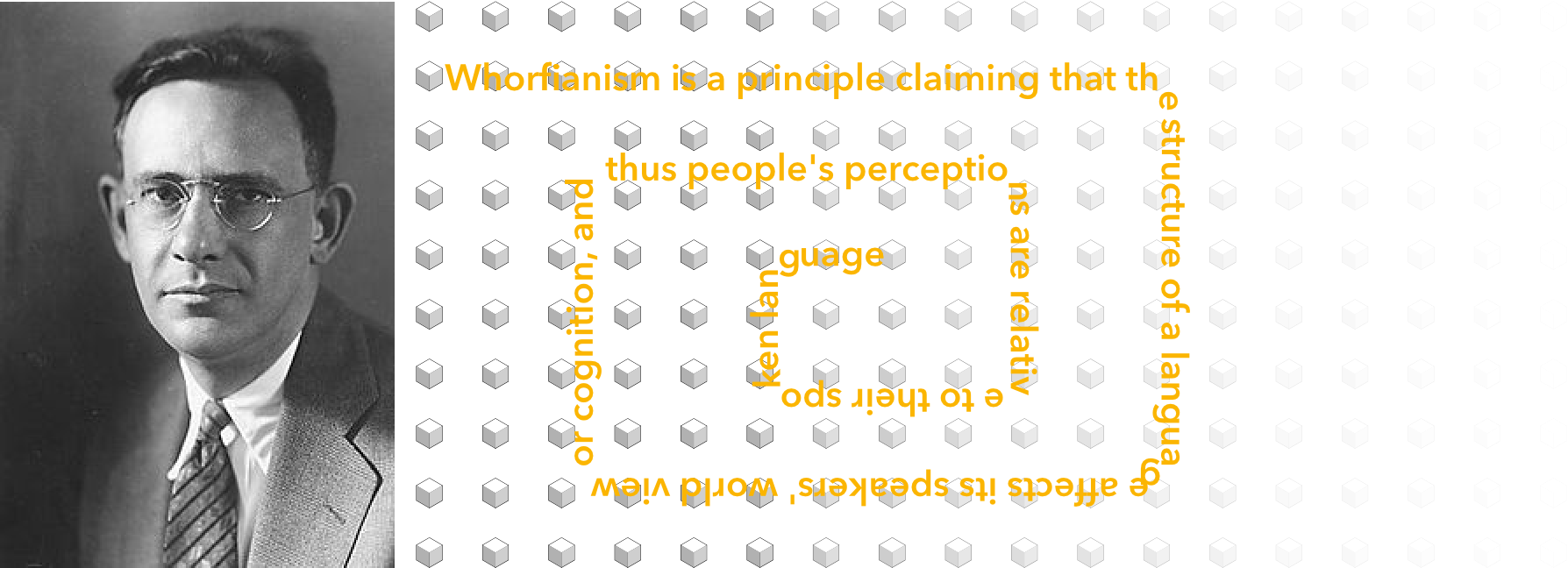# What Are Regular Languages?

Original article was published by Tojo Batsuuri on Deep Learning on Medium# Operations on FSA

We know that Finite State Automata are equivalent to Regular Expressions.

We know that Regular Expressions have operations on them, and sometimes they are closed under these operations.

Similarly, Finite State Automata have operations, and their closure is of interest to us.

Specifically, let us say there are two common languages, L₁ and L₂; there exist automata A₁ and A₂, which accept them.

We know L₁ Union L₂ is also a regular language, so some FSA must exist that accepts it. Question is can you construct this FSA using A₁ and A₂?

## Concatenation

Let A₁ be a finite-state automaton such that L(A₁) = L₁.

Let A₂ be a finite-state automaton such that L(A₂) = L₂.

We then describe an automaton A such that L(A) = L₁ * L₂. A word w is in L₁ * L₂ if and only if it can be broken down into two parts, w₁ and w₂, such that w = w₁*w₂ and w₁ ∈ L₁, w₂ ∈ L2.

This implies that there is an accepting path for w₁ in A₁, and there is an accepting path for w₂ in A₂.

If we allow ϵ-transitions from the final states of A_1 to the initial states of A_2, we can get accepting paths for words of L_1* L_2.

Remember the original definition of FSA.

DEFINITION 1. A finite-state automaton is a five-tuple (Q, q₀, ∑, δ, F) where:

• ∑ is a finite state of alphabet symbols
• Q is a finite set of states
• q₀ ∈ Q is the initial state
• F ⊆ Q is the set of final states, a subset of Q
• δ: Q × ∑ × Q is a relation from states and alphabet symbols to states

We modify this a bit now.

DEFINITION 4. A finite-state automaton A is a five-tuple (Q, q₀, ∑, δ, F) where:

• ∑ is a finite state of alphabet symbols, and is a union of ∑₁ and ∑₂
• Q is a finite set of states and is a union of Q₁ and Q₂
• q₀ ∈ Q is the initial state, specifically q₀ ∈ Q₁
• F ⊆ Q is the set of final states, a subset of Q, specifically F⊆ F₂
• δ: Q × ∑ × Q is a relation from states and alphabet symbols to states, {qf, ϵ, q₀₂ | qf ∈ F₁} where q₀₂ is the initial state of A₂

Remember that regular languages are known to be closed under many operations:

• Intersection
• Complementation
• Exponentiation
• Substitution
• Homomorphism

So it is not surprising that operations on FSA on the same operations are also closed.

## Complementation

If L is a regular language over an alphabet ∑, then the complement of L is the set of all the words from ∑* that are not in L, denoted:

With FSA A such that A = <Q, q₀, ∑, δ, F>, we denote the complement as:

With the understanding that complementation is closed for regular languages, we can show that the intersection of two regular languages is also regular.

## Applications of FSA

We primarily thought of the FSA as a computational device that generates a regular language. However, if we have a word, we can use the FSA to detect the word’s membership in the regular language.

This process takes linear time in the length of the word, regardless of how big the FSA is, this is very attractive. This simple use case is called a dictionary lookup.

It turns out the morphology of most words are simple concatenations of affixes. Since concatenation is closed for regular languages, it makes it easy to implement it with FSA.

Also, the closure properties of FSA help us build models in a modular way.